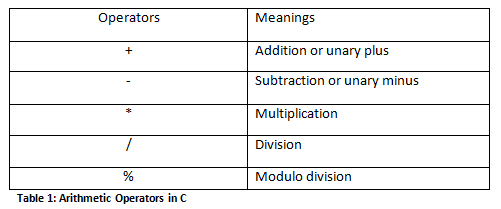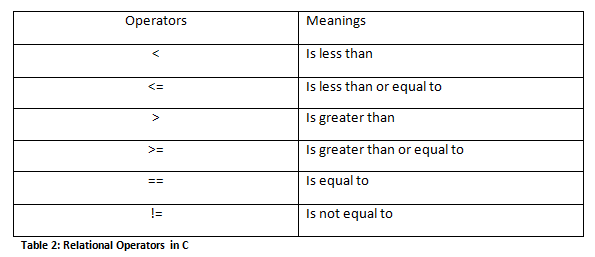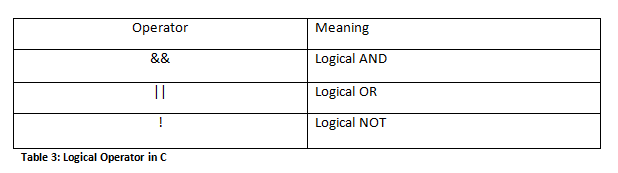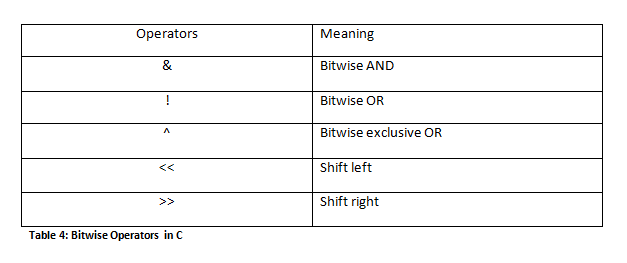# C Programming Operators and Expressions

In this Section, you will learn about Operators in C Programming (all valid operators available in C), expressions (combination of operators, variables and constants) and precedence of operators (which operator has higher priority and which operator has lower priority).

## C Operators

Operators are the symbols which tell the computer to execute certain mathematical or logical operations. A mathematical or logical expression is generally formed with the help of an operator. C programming offers a number of operators which are classified into 8 categories viz.

## 1. Arithmetic Operators

C programming language provides all basic arithmetic operators: +, -, *, / and %.Note: ‘/’ is integer division which only gives integer part as result after division. ‘%’ is modulo division which gives the remainder of integer division as result.

Some examples of arithmetic operators are:

• `a + b`
• `a – b`
• `a * b`
• `a  / b`
• `a % b`

In these examples, a and b are variables and are called operands.

Note: ‘%’ cannot be used on floating data type.

## 2. Relational Operators

Relational operators are used when we have to make comparisons. C programming offers 6 relational operators.Relational expression is an expression which contains the relational operator. Relational operators are most commonly used in decision statements like if, while, etc. Some simple relational expressions are:

• `1 < 5`
• `9 != 8`
• `2 > 1+3`

Note: Arithmetic operators have higher priority than relational operators meaning that if arithmetic expressions are present on two sides of a relational operator then arithmetic expressions will be calculated first and then the result will be compared.

## 3. Logical Operators

Logical operators are used when more than one conditions are to be tested and based on that result, decisions have to be made. C programming offers three logical operators. They are:For example:

`a < 18 || a> 60`

An expression which combines two or more relational expressions is known as logical expression.

Note: Relative precedence of relational and logical operators are as follows

 Highest precedence ! > >= < <= == != && Lowest precedence ||

## 4. Assignment Operators

Assignment operators are used to assign result of an expression to a variable. ‘=’ is the assignment operator in C. Furthermore, C also allows the use of shorthand assignment operators. Shorthand operators take the form:

`var op = exp;`

where var is a variable, op is arithmetic operator, exp is an expression. In this case, ‘op=’ is known as shorthand assignment operator.

The above assignment

`var op = exp;`

is the same as the assignment

`var = var op exp;`

Consider an example:

`x += y;`

Here, the above statement means the same as

`x = x + y;`

Note: Shorthand assignment can be used with all arithmetic operators.

## 5. Increment and Decrement Operators

C programming allows the use of ++ and operators which are increment and decrement operators respectively. Both the increment and decrement operators are unary operators. The increment operator ++ adds 1 to the operand and the decrement operator – subtracts 1 from the operand. The general syntax of these operators are:

Increment Operator: m++ or ++m;

Decrement Operator: m–or –m;

In the example above, m++ simply means m=m+1; and m– simply means m=m-1;

Increment and decrement operators are mostly used in for and while loops.

++m and m++ performs the same operation when they form statements independently but they function differently when they are used in right hand side of an expression.

++m is known as prefix operator and m++ is known as postfix operator. A prefix operator firstly adds 1 to the operand and then the result is assigned to the variable on the left whereas a postfix operator firstly assigns value to the variable on the left and then increases the operand by 1. Same is in the case of decrement operator.

For example,

X=10;
Y=++X;

In this case, the value of X and Y will be 6.

And,

```X=10;
Y=X++;```

In this case, the value of Y will be 10 and the value of X will be 11.

## 6. Conditional Operator

The operator pair “?” and “:” is known as conditional operator. These pair of operators are ternary operators. The general syntax of conditional operator is:

`expression1 ? expression2 : expression3 ;`

This syntax can be understood as a substitute of if else statement.

For example,

```a = 3 ;
b = 5 ;```

Consider an if else statement as:

```if  (a > b)
x = a ;
else
x = b ;```

Now, this if else statement can be written by using conditional operator as:

```x = (a > b) ? a : b ;
```

## 7. Bitwise Operator

In C programming, bitwise operators are used for testing the bits or shifting them left or right. The bitwise operators available in C are:## 8. Special Operators

C programming supports special operators like comma operator, sizeof operator, pointer operators (& and *) and member selection operators (. and ->). The comma operator and sizeof operator are discussed in this section whereas the pointer and member selection operators are discussed in later sections.

#### 1. Comma Operator

The comma operator can be used to link the related expressions together. A comma linked expression is evaluated from left to right and the value of the right most expression is the value of the combined expression.

For example:

`                x = (a = 2, b = 4, a+b)`

In this example, the expression is evaluated from left to right. So at first, variable a is assigned value 2, then variable b is assigned value 4 and then value 6 is assigned to the variable x. Comma operators are commonly used in for loops, while loops, while exchanging values, etc.

#### 2 .Sizeof() operator

The sizeof operator is usually used with an operand which may be variable, constant or a data type qualifier. This operator returns the number of bytes the operand occupies. Sizeof operator is a compile time operator. Some examples of use of sizeof operator are:

```x = sizeof (a);
y = sizeof(float);```

The sizeof operator is usually used to determine the length of arrays and structures when their sizes are not known. It is also used in dynamic memory allocation.

## 9.C Expressions

Arithmetic expression in C is a combination of variables, constants and operators written in a proper syntax. C can easily handle any complex mathematical expressions but these mathematical expressions have to be written in a proper syntax. Some examples of mathematical expressions written in proper syntax of C are:

Note: C does not have any operator for exponentiation.

## 10.C Operator Precedence

At first, the expressions within parenthesis are evaluated. If no parenthesis is present, then the arithmetic expression is evaluated from left to right. There are two priority levels of operators in C.

High priority: * / %
Low priority: + –

The evaluation procedure of an arithmetic expression includes two left to right passes through the entire expression. In the first pass, the high priority operators are applied as they are encountered and in the second pass, low priority operations are applied as they are encountered.

Suppose, we have an arithmetic expression as:

`x = 9 – 12 / 3 + 3 *2 - 1`

This expression is evaluated in two left to right passes as:

First Pass

```Step 1: x = 9-4 + 3 * 2 – 1
Step 2: x = 9 – 4 + 6 – 1

Second Pass

Step 1: x = 5 + 6 – 1
Step 2: x = 11 – 1
Step 3: x = 10```

But when parenthesis is used in the same expression, the order of evaluation gets changed.

For example,

`x = 9 – 12 / (3 + 3) * (2 – 1)`

When parentheses are present then the expression inside the parenthesis are evaluated first from left to right. The expression is now evaluated in three passes as:

First Pass

```Step 1: x = 9 – 12 / 6 * (2 – 1)
Step 2: x= 9 – 12 / 6 * 1```

Second Pass

```Step 1: x= 9 – 2 * 1
Step 2: x = 9 – 2```

Third Pass

`Step 3: x= 7`

There may even arise a case where nested parentheses are present (i.e. parenthesis inside parenthesis). In such case, the expression inside the innermost set of parentheses is evaluated first and then the outer parentheses are evaluated.

For example, we have an expression as:

`x = 9 – ((12 / 3) + 3 * 2) – 1`

The expression is now evaluated as:

First Pass:

```Step 1: x = 9 – (4 + 3 * 2) – 1
Step 2: x= 9 – (4 + 6) – 1
Step 3: x= 9 – 10 -1```

Second Pass

```Step 1: x= - 1 – 1
Step 2: x = -2```

Note: The number of evaluation steps is equal to the number of operators in the arithmetic expression.